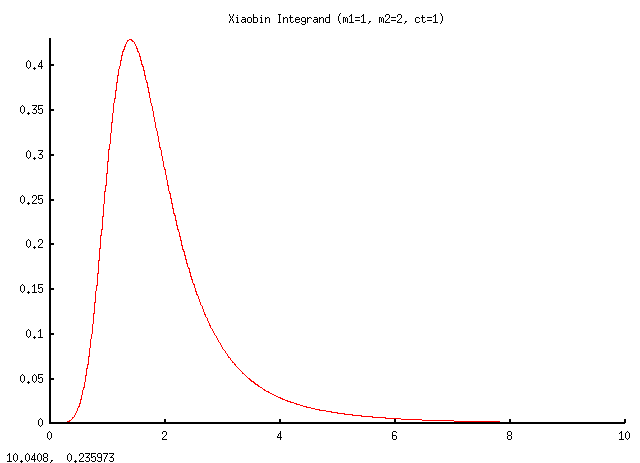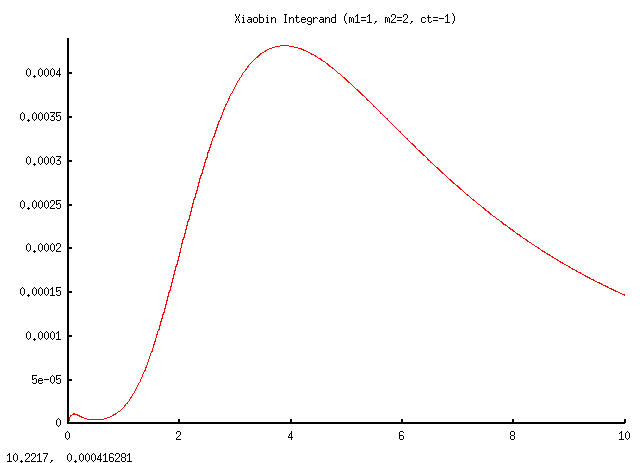# Integral of complicated exponential functions

Wu Xiaobin
I have been working on this integral for a long time ,however i still can't solve it because of the comlicated index of exponential.So if any of you have any idear,please tell me ,thank you so much~~
$$\int _{0}^{\infty }\!{{\rm e}^{-2\,{{\it m1}}^{2}-2\,{{\it m2}}^{2}}}{ \pi }^{-1}r \left( 1+{r}^{2} \right) ^{-3} \left( \left( 1+2\,{{\it m2}}^{2} \right) {r}^{2}+4\,{\it m1}\,{\it m2}\,br+1+2\,{{\it m1}}^{2} \right) {{\rm e}^{{\frac {2\,{{\it m1}}^{2 }+2\,{{\it m2}}^{2}{r}^{2}+4\,{\it m1}\,{\it m2}\,br}{1+{r}^{2}}}}}{dr}$$
I have already tried to substitute r by tan(theta),and i have already refer to the handbook <<Table of integrals,series,and products>>,without finding this type of integral.In the end I have refered to <<Analytical calculation of a class of integrals containing exponential and trigonometric functions>>,howerver i find it to represent results in series of Bessel functions ,that is really not what i want.

Homework Helper
$$\int _{0}^{\infty }\!{{\rm e}^{-2\,{{\it m1}}^{2}-2\,{{\it m2}}^{2}}}{ \pi }^{-1}r \left( 1+{r}^{2} \right) ^{-3} \left( \left( 1+2\,{{\it m2}}^{2} \right) {r}^{2}+4\,{\it m1}\,{\it m2}\,br+1+2\,{{\it m1}}^{2} \right) {{\rm e}^{{\frac {2\,{{\it m1}}^{2 }+2\,{{\it m2}}^{2}{r}^{2}+4\,{\it m1}\,{\it m2}\,br}{1+{r}^{2}}}}}{dr}$$

Good grief that's annoying!

I'd start out by putting
$$P=\frac{e^{-(2m_1^2+2m_2^2)}}{\pi},a=2m_2^2, b=4m_1m_2b, c=2m_1^2$$

I know I defined one b in terms of another b, you can use a different letter if you like...
Then the integral looks something like:

$$P \int_0^{\infty} \frac{r(ar^2+br+c)+r(1+r^2)}{(1+r^2)^3} \exp \left [ \frac{ar^2+br+c}{1+r^2} \right ] dr$$
... provided I haven't stuffed it up. See what I've tried to do?

So if you separate the two parts of the numerator, you get a pair of suggestive looking integrals. (Probably not the word I was looking for...) Looks like you'll be integrating by parts a lot so the derivative of that term inside the exponential will be important.

If I put u(r)=[that stuff inside the exponential] the integrand turns into:

$$\frac{r}{(1+r^2)^2}ue^u + \frac{r}{(1+r^2)^2}e^u$$
... which is still not good but better - and the form is reminding me of something.

That b in the coefficient: 4m1m2b bothers me - without it the quadratic is it part of becomes: $2(m_1+m_2r)^2$

How are you getting on?

Last edited:
Wu Xiaobin
Sorry about replying late.Now I am trying to solve it with your help.And i still takes time to find if it wil do good.Considering the b in the first integrand,it should be cos(theta),and it just make a substitute.

Wu Xiaobin
I have done the integral under your guidance.It seems there still exists the complicated integrand that can't be integrated out after partial integration.

Wu Xiaobin
I have got this eqation,and i wonder if i will help resolve this integral?
$$\int _{0}^{\infty }\!\int _{0}^{\infty }\!{{\rm e}^{-1/2\,{a}^{2}{x}^{ 2}-1/2\,{b}^{2}{y}^{2}-cabxy}}{dx}\,{dy}={\frac {\arccos \left( c \right) }{ab \sqrt{1-{c}^{2}}}}$$

Homework Helper
My own musings have been like to note:

$$\frac{d}{dr}e^u = e^u\frac{du}{dr}$$

and

$$\frac{du}{dr} = \frac{2ar+b}{1+r^2} - \frac{2r}{1+r^2}u$$

this could be one of those messy ones where you have to integrate by parts several times and it turns out that part of the mess is the original integral.

Also there's:
... for integrating quotients, and since we have a square function in the denominator.

Also - this is part of a course? So context within the course will also yield a clue ...

Last edited by a moderator:
Wu Xiaobin
I know what you mean.And i have already done it yesterday.Maybe i should put forward a little further.Since i just did partial integration once.Maybe i would find some relationship between these messy integrand.

Homework Helper
Was this derived from some problem or was it given to you as an exercise?

You may also like to consider an apparently similar problem:
$$\int \exp \left [ x^{(5/2)} \right ] dx$$
The temptation is to do a substitution $u=x^{(5/2)}$
- thing is, there is nothing to absorb the chaff from the transformation to $du=\frac{5}{2}x^{(3/2)}dx$

annoying huh?

If I have something like:
$$\int \frac{r^2}{(1+r^2)^2} \exp \left ( \frac{r^2}{1+r^2} \right ) dr$$
... which is more like what you have.

Here the substitution $u=\frac{r^2}{1+r^2}$ leads to

$$\frac{du}{dr} = \frac{(1+r^2)(2r)-2r(r^2)}{(1+r^2)^2} \Rightarrow du = \frac{2r}{(1+r^2)^2} dr$$
....

But what you have is more like:
$$\int \frac{r^3}{(1+r^2)^3} \exp \left ( \frac{r^2}{1+r^2} \right ) dr$$
... right?

Mathematica refuses to evaluate this one, saying that maybe the integral does not exist. (someone with mathematica or maple could have a better go than I did though.)

But plot it and you see the integral should exist and converge - the actual plot is so sane it's almost boring.

Numerically, that last integral from 0-R, in steps of 1/125, gives me

R=30, I=0.67681
R=300, I=0.67954
R=3000, I=0.67957

clearly converging.

It seems to converge quite fast - so maybe representing the integrand as a converging series - a power, taylor, or fourier series expansion?

BTW: that first integral - mathematica expresses in terms of the En-function:

$$-\frac{2x}{5}E_{\frac{3}{5}}(x^{5/2})$$

It may well be that your integral ends up with a similarly abstract representation.

Last edited:
Wu Xiaobin
It is one part of my research in wireless channel property research,not an exercise.Now i am trying to solve it using partial integration.And after some manipulation,it comes another integral:
$$\int _{0}^{\infty }\!{\frac { \left( ar+1/2\,b \right) {{\rm e}^{u}}}{ \left( 1+{r}^{2} \right) ^{2}}}{dr}$$
And i also trying to substitute r by tan(theta),it turns out another integral which i have no idea.
$$\int _{0}^{\pi/2 }\!{{\rm e}^{(a+c)/2}} \left( b/4+ \sqrt{ {a}^{2}/4\,+{b}^{2}/16}\sin \left( 2\,f+d \right) \right) { {\rm e}^{ \sqrt{{b}^{2}/4\,+\left( a-c \right)\, \sin \left( 2\,f +e \right)/2 }}}{df}$$

Homework Helper
Right - since it is derived for another problem, consider turning it into a series. See what the series turns into.

Sometimes it is worth taking the abstract form of the problem to a mathematician. They may recognize it as part of a class of functions.

Though - immediately, I notice that if you split the denominator you get a pair of integrals that look familiar ... one with r/(1+r2)2 in front of the exponential and the other with just 1/(1+r2)2 in front.

Wu Xiaobin
What is your purpose by giving this integral?Does if refer to partial integration to solve this integral?
$$\int \exp \left [ x^{(5/2)} \right ] dx$$
It seems i can't get the result your paste,but i will try to catch the main point in deducing the result.

Wu Xiaobin
Maple can solve this integral:
$$\int \!{r}^{3}{{\rm e}^{{\frac {{r}^{2}}{1+{r}^{2}}}}} \left( 1+{r}^{2 } \right) ^{-3}{dr}$$

Equals:
$$-1/2\,{{\rm e}^{{\frac {{r}^{2}}{1+{r}^{2}}}}} \left( 1+{r}^{2} \right) ^{-1}$$

Homework Helper
When examining a hard problem it is often useful to look at how simpler problems that share characteristics turn out - this one looked like it had similar complications in the usual approach to solving it - considering it lead through the others. As I get closer to the actual problem, I start to understand how the different terms interact.

Have you tried maple on the full form - the one with the P in front in post #2 ?
Do it as an indefinite integral - I suspect it won't work.

Wu Xiaobin
Yeah,Maple can't solve it.I am going forward through partial integration,however still can't make it.

Homework Helper
Have you tried plotting the integrand to see how it behaves?
Seems very well behaved, especially if m1 and m2 have to be positive.

Looks like you should get good results representing it as an infinite series.

I've attached these two examples, using gnu-octave with the m-file following.Code:
% Physical Parameters
m1=1;
m2=2;
ct=1;
P=(1/pi)*exp(-2*(m1*m1+m2*m2));

%coefficients
a=2*m2*m2;
b=4*m1*m2*ct;
c=2*m1*m1;

%r-axis parameters
N=1024;
s=0;
f=10;

%% calculations

r=s:(f-s)/N:f; % r-axis
h=zeros(size(r)); % locate the r axis

u=(a*r.^2 + b*r + c)./(1+r.^2);

v=r./((1+r.^2).^2);

y=P.*(v.*exp(u) + v.*u.*exp(u)); % Integrand

% representation
plot(r,y,"r",r,h,"k");

% tidy up
box
title("Xiaobin Integrand (m1=1, m2=2, ct=1)")

#### Attachments

Wu Xiaobin
I have already plotted to see its trend.And it really looks like good,and it seems that there should be some kind of expression that can represent this integral.Expanding into series to solve this integral wasn't my purpose honestly.I still think there should have some intricate relationship between the integrand and I just can't figure out a way to kick it.I make me messy these days.How i wish i could solve it.